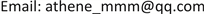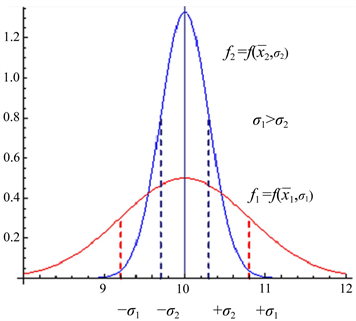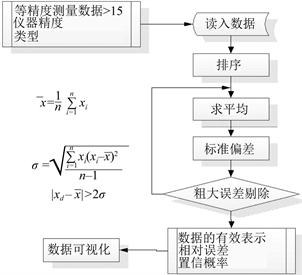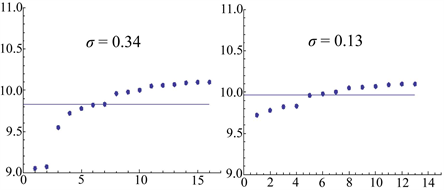﻿ 等精度测量中粗大误差的评估及其在Mathematica上的实现 The Assessment of Gross Error in Equal Precision Measurement and the Fulfillment on Mathematica

Computer Science and Application
Vol. 09  No. 02 ( 2019 ), Article ID: 29075 , 6 pages
10.12677/CSA.2019.92052

The Assessment of Gross Error in Equal Precision Measurement and the Fulfillment on Mathematica

Haizhen Miao

Fujian Jiangxia University College of Information Science and Electronics, Fuzhou FujianReceived: Feb. 10th, 2019; accepted: Feb. 21st, 2019; published: Feb. 28th, 2019ABSTRACT

Since the errors caused by accidental factors are called gross errors, it is very important to determine and eliminate gross errors in data series in equal precision measurement for the best value, uncertainty and confidence probability of measurement results, which will directly affect the best expression of measurement results and the reliability of relative errors. This paper discussed the determination and treatment process of gross error from the probability point of view, and explained how to implement the process on the tools of Mathematica. The conclusion can also be applied to the engineering practice.

Keywords:Equal Precision Measurement, Normal Distribution Function, Standard Deviation, MathematicaCopyright © 2019 by author(s) and Hans Publishers Inc.1. 引言

2. 等精度测量中的测量结果表达式

$f\left(x\right)=\frac{1}{\sigma \sqrt{2\text{π}}}{\text{e}}^{-{\left(x-\stackrel{¯}{x}\right)}^{2}/2{\sigma }^{2}}$

$\sigma =\underset{n\to \infty }{\mathrm{lim}}\sqrt{\frac{\underset{i=1}{\overset{n}{\sum }}{\left({x}_{i}-\stackrel{¯}{x}\right)}^{2}}{n-1}}$ ——正态分布标准偏差

$x=\stackrel{¯}{x}±\Delta$ (单位)

$\stackrel{¯}{x}$ ：直接测量的算术平均值

$\Delta$ ：本次测量的总的不确定度，表明了本次测量的可信程度。大学实验中： $\Delta =\sqrt{{\Delta }_{A}^{2}+{\Delta }_{B}^{2}}$

B类不确定度来自实验中的仪器，不属于本文讨论的范围，A类不确定度来自测量的随机误差，表征就是置信概率，在有限次测量中，该置信概率的计算使用平均值标准偏差，即：

${S}_{\stackrel{¯}{x}}=\frac{\sigma }{\sqrt{n}}=\sqrt{\frac{\underset{i=1}{\overset{n}{\sum }}{\left({x}_{i}-\stackrel{¯}{x}\right)}^{2}}{n\left(n-1\right)}}$

3. 粗大误差对测量的影响

4. 粗大误差的剔除

$|{x}_{i}-\stackrel{¯}{x}|\ge {C}_{n}\sigma$

${x}_{i}$ 被认为是粗大误差。Figure 1. Measured data of equal precision measurement

5. Mathematica简介

Mathematica是由美国科学家斯蒂芬·沃尔夫勒姆领导的沃尔夫勒姆研究公司开发的一款使用的计算软件。它拥有强大的数值计算和符号运算能力。它是目前为止使用最广泛的数学软件之一。广泛地应用于物理学、工程学、数学、生物和社会学等众多领域  。

6. 处理过程及数据可视化Figure 2. Processing procedure

Mean [list]：给出列表list中元素的统计平均值；

Standard Deviation [list]：给出list中元素的标准差；

Variance [list]：给出list中元素的样本方差；

Normal Distribution [ $\mu$ , $\sigma$ ]：表示一个正态分布(高斯分布)，它的均值为 $\mu$ ，标准差为 $\sigma$  ；

PDF [dist, x]：给出关于在 $x$ 处的符号分布dist的概率密度函数  ；

$\text{Plot}\left[f,\left\{x,\text{Subscript}\left[x,\mathrm{min}\right],\text{Subscript}\left[x,\mathrm{max}\right]\right\}\right]$ ：绘制函数 $f$ 的图线，其自变量 $x$ 位于从 $\text{Subscript}\left[x,\mathrm{min}\right]$$\text{Subscript}\left[x,\mathrm{max}\right]$ 的区间上  。

(*剔除粗大误差*)

While[True,

If [data=Abs[Min[datalist]-databest]>2*datasd,{datalist=Delete[datalist,1],

datalenth=Length[datalist],

databest=Mean[datalist],

datasd=StandardDeviation[datalist]},y=1];

If[y==1,Break[]]

While[True,

If [data=Abs[Max[datalist]-databest]>2*datasd,{datalist=Delete[datalist,-1],

datalenth=Length[datalist],

databest=Mean[datalist],

datasd=StandardDeviation[datalist]},x=1];

(*未剔除粗大误差*)

(*剔除粗大误差*)

A类不确定度：0.04Figure 3. Precision without considering gross errors precision after eliminating gross error

7. 在工程实践中的应用

8. 结论

The Assessment of Gross Error in Equal Precision Measurement and the Fulfillment on Mathematica[J]. 计算机科学与应用, 2019, 09(02): 458-463. https://doi.org/10.12677/CSA.2019.92052

1. 1. 李汉龙, 主编. Mathematica基础及其在数学建模中的应用[M]. 北京: 国防工业出版社, 2016.

2. 2. Grozin, A. (2015) Introduc-tion to Mathematica® for Physicists. Springer, Softcover Reprint of the Original.

3. 3. Wolfram, S. (2016) An Elementary Introduction to the Wolfram Language. Wolfram Media Inc.

4. 4. Napolitano, J. (2018) A Mathematica Primer for Physicists. CRC Press, ‎Boca Raton.

5. 5. (美)克里夫•黑斯廷斯, 等. Mathematica实用编程指南[M]. 北京: 科学出版社, 2018.